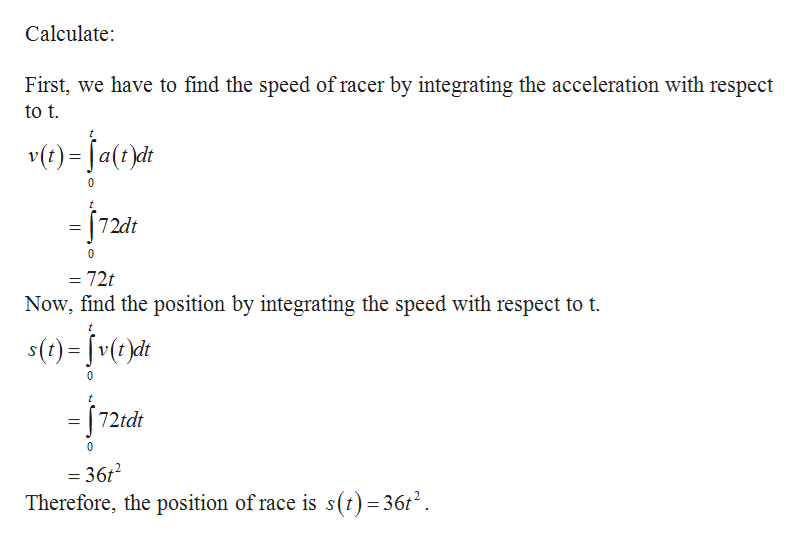# A drag racer accelerates at​a(t)equals=7272​ft/ssquared2.Assume that​v(0)equals=0and​s(0)equals=0. a. Determine the position function fortgreater than or equals≥0.b. How far does the racer travel in the first66​s?c. At this​ rate, how long will it take the racer to travelone third13​mi?d. How long will it take the racer to travel300300​ft?e. How far has the racer traveled when it reaches a speed of183183​ft/s?

Question
2 views
A drag racer accelerates at
​a(t)equals=7272
​ft/ssquared2.
Assume that
​v(0)equals=0
and
​s(0)equals=0.

a. Determine the position function for
tgreater than or equals≥0.
b. How far does the racer travel in the first
66
​s?
c. At this​ rate, how long will it take the racer to travel
one third13
​mi?
d. How long will it take the racer to travel
300300
​ft?
e. How far has the racer traveled when it reaches a speed of
183183
​ft/s?
check_circle

Step 1

(a)

Given information:

A drag racer accelerates at a(t)...help_outlineImage TranscriptioncloseCalculate: First, we have to find the speed of racer by integrating the acceleration with respect to t v(t)=Ja(t)d -72dt 72t Now, find the position by integrating the speed with respect to t t s()=v(t)dt 0 72tdt 0 = 36t Therefore, the position of race is s(t) =36t2 fullscreen

### Want to see the full answer?

See Solution

#### Want to see this answer and more?

Solutions are written by subject experts who are available 24/7. Questions are typically answered within 1 hour.*

See Solution
*Response times may vary by subject and question.
Tagged in

### Calculus